International Journal of Geomagnetism and Aeronomy
Vol 2, No. 1, June 2000

# IMF length scales and predictability: The two length scale medium

Michael R. Collier, Adam Szabo, James A. Slavin, and R. P. Lepping

NASA/Goddard Space Flight Center, Laboratory for Extraterrestrial Physics, Greenbelt, Maryland

S. Kokubun

Solar-Terrestrial Environment Laboratory, Nagoya University, Toyokawa, Japan

### Abstract

We present preliminary results from a systematic study using simultaneous data from three spacecraft, Wind, IMP 8 and Geotail to examine interplanetary length scales and their implications on predictability for magnetic field parcels in the typical solar wind. Time periods were selected when all three spacecraft were within one Earth radius of residing in a plane that includes the GSE x direction so that if the parcel fronts were strictly planar at some angle relative to the Sun-Earth line and radially convecting, the two adjacent spacecraft pairs would determine the same phase front angles. After correcting for the motion of the Earth relative to the interplanetary medium and deviations in the solar wind flow from radial, we used differences in the measured front angle between the two spacecraft pairs to determine structure radius of curvature. Results indicate that the typical radius of curvature for these IMF parcels is of the order of 100  RE. This implies that there are two important IMF scale lengths relevant to predictability: (1) the well-established scale length over which correlations observed by two spacecraft decay along a given IMF parcel, of the order of a few tens of Earth radii and (2) the scale length over which two spacecraft are unlikely to even observe the same parcel because of its curvature, of the order of a hundred Earth radii.

### Introduction

Studies concentrating on solar wind-magnetosphere interaction frequently and routinely use upstream monitors to determine how changes in solar wind properties affect the magnetosphere. Not uncommonly, the only remark made is that an "upstream monitor" was employed as if location upstream were completely irrelevant. There appears to be a lack of appreciation of the interplanetary medium as a structured, as opposed to homogeneous, entity.

Yet Sun-Earth connection studies which aim to determine how accurately an upstream monitor can predict the solar wind parameters that actually interact with the magnetosphere (or even reach the outer heliosphere [Sibeck and Richardson, 1997]) are showing that the interplanetary medium is not uniform over magnetospheric scale lengths. Because the scale length of the geoeffective flow is only a few Earth radii around the Sun-Earth line [Burke et al., 1999], the trick to predicting geoeffectiveness is to determine what observations at the upstream spacecraft location reveal about conditions within a couple Earth radii of the Sun-Earth line, and this may be inferred by using observations from multiple spacecraft.

It should be pointed out that these types of studies have importance beyond solar wind-magnetosphere interactions. They apply, for example, to bow shock modeling [Safránková et al., 1999]. Even interplanetary multi-spacecraft studies using one spacecraft for "baseline" solar wind must have an appreciation for scale lengths and coherency within the interplanetary medium to evaluate baseline accuracy [Owen et al., 1996].

### 2. Comments on the Two Spacecraft Study of Collier et al. 

This paper elaborates and extends the two spacecraft work described in Collier et al.  which employed Wind and IMP 8 magnetic field data. In their correlation study, they restricted attention to those cases exhibiting good correlation, which was defined as cases having a peak Crooker hybrid correlation coefficient above 0.8 [Crooker et al., 1982]. This was done because most cases had peak correlation coefficients in the range 0.5-0.8 so that, for example, evaluating an average peak Crooker hybrid correlation coefficient would show little effect and have large dispersions.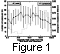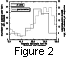Nevertheless, we can learn something by examining all cases together. Figure 1 shows for all of the two hour data intervals studied in Collier et al. , the angular distribution of the magnetic field direction and how the average peak Crooker hybrid correlation varies with the average magnetic field direction projected into the ecliptic plane. The solid data points and line show the average correlation for all 543 cases (not just the good correlation cases) falling within that 10o bin. The minimum average peak Crooker hybrid correlation coefficient in a 10o bin is 0.519 and the maximum is 0.710, so that the difference between the minimum and maximum average peak Crooker hybrid correlation coefficient in a 10o bin is only 0.191, close to the typical scatter in the data ( 1s ), indicated by the error bars, so some might argue this effect is not convincing. (However, Figure 2 which will be discussed in detail below shows that most cases of good correlation are around 90o as compared to those at the Parker spiral direction.)

Thus there is a clear tendency for higher correlation to be observed when the IMF is perpendicular to the Sun-Earth line, consistent with the findings of Crooker et al.  who deduced that magnetic features are correlated a longer distance along the field than perpendicular to the field. This implies better correlation when the field is perpendicular to the Sun-Earth line because in this case the same field line will intersect both spacecraft but at different locations along the field line. When the field line is parallel or anti-parallel to the Sun-Earth line, the two spacecraft sit on different field lines and are separated solely perpendicular to the field resulting in lower correlation. However, as discussed above, the effect is not that large.

This may provide an explanation for why studies have typically found that the optimal assumed angle for propagation is neither perpendicular to the Sun-Earth line nor along the Parker spiral, but rather between the two. For example, Richardson and Paularena  found ~ 115o for plasma structures [see also Paularena et al., 1998; Richardson et al., 1998]. They interpret these results as indicating that time-dependent changes in the solar wind source are as important as longitudinal variations of the source. Here we provide an alternative explanation.

The dashed lines in both Figure 1 and 2 show the probability distribution for the IMF direction, which is, of course, peaked along the Parker spiral direction, 135o, with a minimum, although non-negligible, probability of having a two-hour averaged field direction in the ortho-Parker direction, 45o. Non-standard field directions are attributed to large amplitude waves, discontinuities, convected structures and variations in flow speed but in some cases could indicate the presence of unusual field configurations at the solar wind source, at a few solar radii, where it has been usually assumed that field lines are near radial [Jones et al., 1998]. The angular distribution of good correlation cases, those cases which can be reliably employed to establish timing, will depend on two factors: (i) the distribution of the average correlation coefficients (the solid line in Figure 1) because, all things being equal, good correlation cases will most likely occur at the angle where the average correlation coefficient is maximum, 90o, and (ii) the distribution of field angles (the dashed line in Figure 1) because, again all things being equal, the more cases with the IMF at a given angle (e.g. 135o, the Parker spiral direction), the more likely a good correlation case will occur at that angle. Consequently, we expect the distribution of good correlation cases to peak somewhere between 90o and 135o, a compromise between the two distributions.

Indeed, Figure 2 shows with the solid line the direction probability distribution for the 67 cases with good correlation, an average peak Crooker hybrid correlation coefficient above 0.80. Here, the bins are 30o wide and the average is 106.3o, as expected, a compromise between perpendicular to the Sun-Earth line and the Parker spiral direction.

This may also explain, when the large width of the distribution is also considered, why neither convection nor corotation shifting appears to be superior for timing purposes [Couzens and King, 1986; Kelly et al., 1986; King, 1986].

### 3. Possible GSE x-DependenceIn agreement with the conclusions of Lyons et al.  and of Silverman et al.  for SOHO/Wind plasma data correlations, we do not find any convincing evidence of an effect due to spacecraft separation in the GSE x -direction (parallel to the solar wind flow direction) in the correlation results. In an attempt to isolate the effects of parallel spacecraft separation from perpendicular spacecraft separation, we examined those cases of the 543 Wind/IMP 8 two hour data intervals in which the spacecraft were separated by 50-60 Earth radii perpendicular to the Sun-Earth line. This interval was picked to maximize the parallel range and the case statistics. Figure 3 shows the average Crooker hybrid correlation coefficient as a function of spacecraft separation in the GSE x -direction for a fixed perpendicular separation of 50-60 Earth radii. Although the x -separation spans nearly a factor of two, from 140 to 260  RE, there is no apparent change in the average correlation coefficient.

### 4. A Simple Timing Model

If we assume the distribution of parcel front angles (see [Collier et al., 1998, Figure 3]) is wide enough to be approximated by a uniform distribution, we can find an analytic expression for the distribution of lag times. Note that by parcel front, we mean the direction along which the correlation between the magnetic field observed at two different points is greatest. Parcel fronts are lines of maximum correlation. If their angular distribution is uniform, then the probability of a given phase front being at a particular angle is simply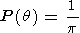(1)

Simple trigonometry shows that if the spacecraft are separated by distances dpar and dper parallel and perpendicular to the Sun-Earth line, respectively, then(2)

where vsw is the solar wind speed. Given that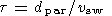(3)

this can be re-written as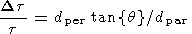(4)

where Dt is the difference between the observed and the convection lag times. Thus, we can write an expression for q :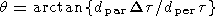(5)

To determine what this says about the distribution of lag times, we use the expression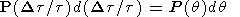(6)

which means simply that, using P(q) from (1) above,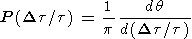(7)

Evaluating this expression using (5) above results in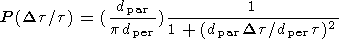(8)

which is a Cauchy distribution. Note that this distribution has no mean or standard deviation (the integrals diverge) resulting in some counter-intuitive properties.

### 5. Comment on Cauchy Flight Model

One example is that an attempt to determine an average Dt/t from a distribution of this type results in a Lévy flight process and, more specifically, a Cauchy flight [Collier, 1993a, 1993b] due to the power law nature of the tail. Whereas normally s/N where s is the standard deviation and N is the number of events decreases as 1/N1/2, for a Cauchy flight process, s/N does not decrease with N (i.e. sN ). Thus, increasing the number of measurements will not increase the accuracy of the measurement. Fundamentally, this is due to the high probability of very bad timing errors resulting from the tails "throwing off" the averaging process. However, it raises the intriguing possibility that the accuracy in determining convection times using two upstream observations will not improve with multiple measurements - that is there will be the same uncertainty whether one or a thousand measurements are made [Schroeder, 1991].

### 6. Short Scale Length (~10s of RE)

It has become well-established that the scale length over which correlation decays along a solar wind parcel is of the order many tens of Earth radii. Table 1 lists six studies, all of which applied different techniques, which support a scale length of many tens of Earth radii, particular values ranging from ~10 to 60, depending on the details of the study.

Crooker et al.  using ISEE 1 and ISEE 3 data showed that during periods of low IMF variance good correlations tend to occur when the distance between the two spacecraft perpendicular to the IMF is less than 20  RE. However, during periods of high variance the scale size is larger, about 50  RE. Borodkova et al.  employed Prognoz 10 as a solar wind monitor for periods when transient variations in the dayside magnetospheric magnetic field were observed by GOES 5 and 6 geosynchronous satellites. They found a decrease in the success rate for associating the transient events with solar wind features as Prognoz 10 moved farther off the Sun-Earth line. The decrease has a scale length of the order of a few tens of Earth radii based on their Table 2. Lyons et al.  used Wind, IMP 8 and Geotail data along with CANOPUS meridian-scanning photometer data to investigate the IMF behavior relating to substorm onsets. They found that a clear IMF trigger, a northward turning or a reduction in the magnitude of the y component, could be identified in 14 of the 20 substorms considered and the probability of trigger identification is a strong function of IMF monitor distance from the Sun-Earth line: 89% for monitors that are within 30  RE of the Sun-Earth line but only 50% for monitors located 30-56.7  RE from the Sun-Earth line. Sotirelis et al.  used polar rain observations from DMSP along with IMF observations from IMP 8 to show that a solar wind monitor greater than 10  RE from the Sun-Earth line has a 20% chance of seeing a significantly different IMF than that impinging on the magnetosphere. Collier et al.  using IMF data from Wind and IMP 8 showed that the probability of obtaining a good correlation between observations made off the Sun-Earth line and those along the Sun-Earth line is an exponential-like function of the monitor distance from the Sun-Earth line with an e -folding distance of about 40  RE. Finally, Kessel et al.  used Geotail, Wind and IMP 8 data to examine a two hour time period on September 14, 1995 when Geotail on the dawnside measured magnetic field directions 60-120 degrees different from those measured by Wind on the duskside ( y -GSE separation of 60  RE ). Kessel et al. suggest there is a kink in the magnetic field near the Sun-Earth line and, insofar as the inference is geometrical, this study may be more pertinent to the longer, curvature, scale length to be discussed next, although in size it appears to fall into the short scale length category.

The sum total of these studies indicates that the IMF has one (short) scale length of the order of many tens of Earth radii (although the Kessel et al.  study may be more pertinent to the longer scale length to be discussed in the next section).

### 7. Long Scale Length (~100 RE)

It is the contention of this paper that in addition to the short scale length of the order of many 10s of RE representing correlation decay along a parcel there is a longer scale length of the order of 100  RE representing large scale geometry.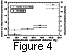Previous work by Crooker et al.  using ISEE 1 and ISEE 3 data hinted at the presence of this longer scale length. The solid circular data points, corresponding to the left hand y axis, on Figure 4 show a replotting of the data shown in the two panels of Figure 2 of Crooker et al. . The ratio of the number of cases with poor correlation (less than 0.5) to the number of cases with good correlation (greater than 0.8) increases abruptly at around 100 Earth radii. We have verified the Crooker et al. results with a similar approach using Wind and IMP 8 data as shown with the square data points and right hand y axis in Figure 4. Note that the left and right y axis scales are different because we are emphasizing the similar qualitative behavior in the two studies. Although we see fewer good cases, perhaps because of solar cycle effects, the same jump observed by Crooker et al. at around 100  RE is apparent in our data as well.

One possible physical interpretation of these data, corroborated by the work reported here, is that interplanetary parcels have a characteristic radius of curvature of about 100  RE, and beyond this perpendicular spacecraft separation, the two spacecraft will frequently not even observe the same field parcel. Hence the dramatic increase in poor correlation cases which indicates little or no similarity in the observations at the two spacecraft. This should be contrasted with the shorter scale length which represents a decay in the probability of a good correlation and is interpreted as a coherency scale length along a single parcel.

Much of the analysis performed examining interplanetary parcels has assumed planar structure fronts, and one purpose of this study is to determine how reasonable this assumption is, that is, what is the characteristic radius of curvature. However, one difficulty is that, if the structures are perfectly planar, the orientation is determined by two angles, for example azimuth and elevation, and two arbitrarily placed spacecraft will only determine one angle, leaving the other undetermined. To check this determined angle for consistency with another spacecraft and infer a radius of curvature, the second spacecraft pair must be placed geometrically so that the measured angle is the same angle. A little reflection makes it clear that this means the plane formed by the three spacecraft must include the solar wind flow direction, assumed for this purpose to be the GSE x direction.

In principal, one could perform studies investigating the radius of curvature both in and out of the ecliptic plane; one would not generally expect them to be the same. In practice, determining radii of curvature out of the ecliptic is complicated for two reasons: (i) The spacecraft move with the Earth at 17  RE per hour and the convection times from Wind to IMP 8 and Geotail are frequently of the order of an hour. Consequently, looking for time periods when the plane formed by the three spacecraft includes the GSE x axis must consider and account for the observed parcel lag times which are not known a priori. This is in contrast to determining curvature in the ecliptic plane where this effect only changes the effective perpendicular separation between the spacecraft but not their immersion in the plane. (ii) The spacecraft are usually close to the ecliptic plane so it is difficult to get large spacecraft separations perpendicular to the ecliptic plane. Consequently, the radius of curvatures determined here are effectively in the ecliptic plane.

### 9. Selection Criteria

To be considered a valid case for analysis, a number of conditions had to be met. First, all three spacecraft had to be within one Earth radii of residing in a plane (close to the ecliptic) that includes the GSE x -direction, the solar wind flow direction, and be well-separated in the GSE y direction. One Earth radius may reflect the accuracy with which IMP 8's position is currently known. The times when this first condition was satisfied were determined using data from the Satellite Situation Center (http://sscweb.gsfc.nasa.gov/).

These "triplanarity" time periods are determined by assuming the solar wind flows along the x axis and are given a 1  RE tolerance. The error introduced by the 1  RE tolerance depends on structure angle out of (i.e. perpendicular to) the plane which is assumed not so steep that this will be a significant source of error.

As mentioned earlier, the triplanarity condition assures that both spacecraft pairs observe the same angle. The well-separated condition was imposed to make sure that the angles were well-determined, which requires a wide perpendicular spacecraft separation (of course, correlation also gets worse with increasing perpendicular separation). Quantitatively, we required that each pair of adjacent spacecraft have a perpendicular separation of at least five Earth radii.

Second, all three spacecraft had to be in the interplanetary medium. For Wind, which was usually far upstream, this was not an issue. For IMP 8, we used the solar wind speeds from MIT (ftp://space.mit.edu/pub/plasma/imp/www/imp.html), which are determined only when the spacecraft is in the interplanetary medium. To assure that the data had no sheath periods in it, we took care to make sure no one hour intervals adjacent to the bow shock were used [D. G. Sibeck, private communication, 1999].

For Geotail, we examined the CPI spectrograms (http://www-pi.physics.uiowa.edu/www/cpi/) to assure that Geotail was in the solar wind [Frank et al., 1994]. Geotail was used to establish the even hour interval used which has good correlation (e.g. 2000-2100) with the corresponding data intervals for Wind and IMP 8 shifted according to the observed lag time.

Third, all three of the two-spacecraft pairs had to have clear peaks in the average correlation coefficient as a function of lag time to assure the convection lag times are well-defined. The average correlation coefficient (average of the three components and magnitude) is more convenient than the Crooker hybrid and mimics its behavior closely, particularly near the peaks [e.g. Collier et al., 1998; Kaymaz and Sibeck, 1999, 2000]. The resultant lag times were then checked for consistency between the three spacecraft by requiring that the lag time between upstream spacecraft A and B plus the lag time between upstream spacecraft B and C equal the lag time between upstream spacecraft A and C to within 72 seconds (0.02 hours - reasonable uncertainty due to the one minute averaging time) as it must if all spacecraft were observing the same parcel. This is a strong check in part because all three lag times are determined separately.

If the parcel is static and undergoes no temporal evolution, then the sum of the lag times between the upper and lower spacecraft pairs must equal the lag time between the two outside spacecraft, regardless of parcel geometry. Thus, the fact that we were not able to find a case with well-defined lag times that violates this condition supports the inference that there is little temporal evolution, i.e. GSE-x dependence, that these parcels undergo.

The magnetic field data from all potential cases were carefully examined for possible foreshock and sheath contamination [Berdichevsky et al., 1999; Zastenker et al., 1999], as it has been established that foreshock and sheath effects adversely affect correlation [Kaymaz and Sibeck, 1999, 2000]. We assured that there was no evidence of foreshock contamination by looking for turbulent signatures in the magnetic field data. This was, of course, only an issue for Geotail and IMP 8.

### 10. Corrections to dper and dpar

Because it is frequently the case that the observed angles based on two spacecraft pairs are frequently very close, it is important to correct the spacecraft distances for two effects: (i) the solar wind flow angle and (ii) the motion of the Earth around the Sun. Both effects will modify the effective perpendicular spacecraft separation, and the solar wind flow angle will modify the effective parallel spacecraft separation.

The solar wind flow direction was determined using Wind SWE (96 second averages) data averaged over the relevant one hour interval shifted for the Wind-Geotail lag time to determine an angle b the solar wind flow forms with the GSE x axis. The perpendicular and parallel spacecraft separations dper and dpar respectively were then modified by the following expressions:(9)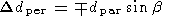(10)

which is simply the small angle approximation of the standard rotation matrix with the appropriate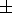combination selected on the basis of geometry.

We incorporated the motion of the Earth simply by modifying the perpendicular spacecraft separation for each pair by an amount dependent on the observed lag time between the two spacecraft and the speed of the Earth (30 km s -1 or about 17  RE /hour) with the sign dependent on the relative spacecraft locations.

### 11. Calculating the Curvature of Interplanetary Parcels

Using the observed solar wind lag times between each spacecraft pair, tobs, the solar wind convection time t (determined using Wind SWE data over the appropriate time period inferred by the observed Wind-Geotail lag time), and the perpendicular and parallel spacecraft separations, dpar and dper respectively, one can determine the front angle q using the relationship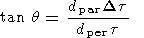(11)

where Dt = t - tobs. This is just equation (4).

By obtaining two parcel angles, one for the "lower" (in a GSE y sense) and one for the "upper" spacecraft pair ( q1 and q2 respectively), one may use the expression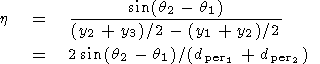(12)

to determine the curvature, h. Here dper1 and dper2 are the perpendicular separations of the lower and upper spacecraft pairs, respectively.

The reciprocal of the curvature, h, yields the radius of curvature r, which is a more physically intuitive quantity. We elect, however, to perform the analysis using h because frequently the results are consistent with planar parcels which imply zero curvature but infinite radius of curvature.

### 12. Example Cases: August 23, 1995, 2000-2100, and September 2, 1996, 0200-0300

We have canvassed all of 1996 and about half of 1995 looking for valid time periods as described above. So far, we have eight listed in Table 2.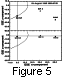Figure 5 shows an example of a time period when the plane formed by the three spacecraft includes the GSE x axis; Wind, IMP 8 and Geotail are only a couple of RE below the ecliptic plane. As shown in the upper panel, the spacecraft geometry is well-suited for a determination of structure curvature in that the spacecraft are reasonably well-separated perpendicular to the Sun-Earth line.

Although Geotail is close to the nominal bow shock position, indicated by the solid line shaped like the bow shock, CPI data from Geotail indicate that it was in the solar wind at this time.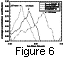Figure 6 shows the cross correlation as a function of lag time for the three spacecraft pairs, Geotail/IMP 8, Geotail/Wind and Wind/IMP 8. What is plotted here at each lag time is an average correlation coefficient which represents the average of the correlation coefficients for the magnitude and the three components of the magnetic field. Note that the lag time from Wind to IMP 8 (0.15 hours) plus the lag time from IMP 8 to Geotail (0.13 hours) is equal to the lag time from Wind to Geotail (0.27 hours) to within uncertainty, as it must be if the parcels are coherent and time-stationary.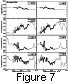Figure 7 shows a comparison between magnetic field magnitudes and the GSE x, y, and z components for both Geotail and IMP 8 (left column) and Wind and IMP 8 (right column). In these figures, the IMP 8 time tags have been adjusted by the observed lag time to line up the data.

One common misconception about correlation studies is that the technique will focus on one or a few discontinuities in the data set so that, for example, parcel fronts could be determined by a variance analysis [Sonnerup and Cahill, 1967]. As is apparent from the figure, this is not the case. Instead, the technique does seem to try to match the entire field profile over the time interval without matching up a single feature, an important point to remember when interpreting these studies: the parcel front angle does actually represent the entire parcel and not a particular discontinuity within the parcel. It is simply the case that the amplitudes of the discontinuities are usually much too small to dominate the total variance. Of course, if one focuses specifically on discontinuities, it may be possible to determine the timing of these particular structures with greater accuracy [e.g. Khan and Cowley, 1999].

It is also interesting that one can see clear spatial structure that varies over the spacecraft perpendicular separation. For example, the spike in the Geotail y component at Geotail hour 20.3 is larger at Wind and smaller at IMP 8, the two spacecraft straddling Geotail in the y -GSE direction. Thus, this spike systematically rises in magnitude from about 1 nT at IMP 8 to about 4 nT at Wind over a perpendicular distance of twenty or so RE. Note from Figure 5 that Geotail is closer in a GSE- y sense to Wind than to IMP 8 and the peak average correlation coefficient, as shown in Figure 6, is the greatest of the three pairs. This is consistent with the correlation being dependent on perpendicular spacecraft separation. It should also be pointed out that the Wind-Geotail correlation is greatest in spite of the largest GSE- x separation of the three pairs, also consistent with a smaller or negligible dependence of correlation on GSE- x separation. Finally, the spike at Geotail hour 20.3 is closer in magnitude to that observed at Wind than that at IMP 8, suggesting not counter-intuitively that not only correlation, that is changes, but also the agreement of actual magnetic field values is dependent on perpendicular spacecraft separation.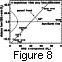It is interesting to note that it is not unusual to find time periods when the spacecraft farther upstream actually observes the parcel later than the downstream spacecraft due to the orientation of the parcel front. A couple such time periods occur in the list in Table 2, and the location of the spacecraft along with the orientation of the parcel front is shown in Figure 8 for one of these time periods on September 2, 1996, 0200-0300. In this case, the straight convection time (which assumes the front lies perpendicular to the Sun-Earth line) would have IMP 8 observe the parcel 0.035 hours after Geotail. Instead, IMP 8 observes the parcel 0.02 hours before Geotail implying a front angle of about 53o, as indicated in Figure 8, and corroborated by the Wind/Geotail analysis.Table 2 column 6 summarizes the curvature results from the eight cases we have examined so far. Many of the cases are consistent with no curvature, that is perfectly planar parcels. The curvature for each case is plotted in Figure 9. The average curvature is 8.510-3 RE-1, implying a typical radius of curvature of 118  RE. Here we quote unweighted values because weighted values would attach too much importance to infrequent, well-determined outliers.

### 14. Conclusion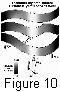Figure 10 shows the picture of interplanetary parcels that appears to be emerging. These parcels are convected approximately with the solar wind speed with little or no temporal evolution over a couple hundred or less earth radii. When observing the same parcel two spacecraft have a correlation scale length of about 30 Earth radii indicated by the gray scale in Figure 10. This scale length is not to be confused with the curvature scale length, and although the scale length is shown the same on all parcels, there is certainly variability from parcel to parcel. This means that a spacecraft's ability to determine the characteristics of the parcel of the interplanetary medium that impacts the magnetosphere will decay with distance from the Sun-Earth line with a characteristic e -folding length of about 30 Earth radii in accordance with this work and the studies listed in Table 1. This is the effect illustrated by the gray scale in Figure 10 and is why the parcels are white in the center and black at the edges.

However, these parcels also have a radius of curvature of the order of 100 Earth radii indicated by the curved boundaries of the parcels in Figure 10. Thus, at a characteristic separation of about a hundred Earth radii, two spacecraft will frequently not even observe the same field profile, as evidenced by a dramatic increase in poor correlation cases observed by Crooker et al.  and in this study (Figure 3). This means that the critical question when trying to determine what impacts the Earth based on observations at distances from the Sun-Earth line of the order of 100  RE is not how well the observations predict what is happening along the Sun-Earth line, but rather whether there is any relationship whatsoever between the spacecraft observations and what impacts the Earth. Because our observations show that the parcel radius of curvature is ~100  RE which is usually significantly larger than the typical separation between the spacecraft perpendicular to the Sun-Earth line, planarity is, in most cases, a reasonable assumption.

In principal, there could be a bias in the results due to radii of curvature being smaller than the typical interspacecraft separation. This would bias the results toward larger radii of curvature because shorter ones would be missed. However, this is probably not a significant effect because we expect the distribution of radii of curvature to be relatively well-behaved. Consequently, if we were missing a significant number of parcels with shorter radii of curvature due to interspacecraft separation effects, we would expect to observe quite a few cases with radii of curvature of the order of the perpendicular spacecraft separations. Yet, the shortest radius of curvature observed is 46  RE with seven of eight cases greater than 79  RE, and the average is 118  RE. Thus, it is unlikely that we are missing many parcels with short radii of curvature.

Correlation studies such as these described herein have a practical aim: to help us predict space weather. However, because it appears that the basic morphology of the solar wind is determined very close to the Sun [Breen et al., 1999], such studies may illuminate various solar processes. So, given the existence of a correlation scale length of many 10s of RE and a geometric scale length of ~100  RE, a natural question to ask is what processes in the solar corona or interplanetary medium would produce these scale lengths.

One possibility is that large scale effects are created by very small scale structures too minute to observe. However, evidence suggests that it might be possible to associate discrete structures seen in the solar wind with specific details of the solar atmosphere [Fisher and Guhathakurta, 1995]. Also, Roberts and Ghosh  have raised the interesting possibility that the parcel phase fronts and curvature may be due to velocity shear in the solar wind.

Furthermore, the average solar wind speed for the eight cases examined in this study is 377 km s -1, which is lower than the average solar wind speed. This is consistent with Chang and Nishida's  observation that lower solar wind speeds are associated with higher correlation. However, lower speed solar wind tends to be more turbulent, more of a "garbage" wind that is not as well-behaved as the higher speed solar wind, and it is significant that the typical radius of curvature is still large, of the order of 100  RE. A difference in the radius of curvature of solar wind parcels between slow and fast wind could be linked to the origin of the slow wind on the solar surface.

Finally, if the 100  RE scale length is extrapolated back radially (214  R<IMG SRC='latex032.gif' ALT="odot"> ) to the solar surface, one gets a scale length of about 3000 km. However, it is likely that super-radial expansion occurs, so that the actual scale length will be slightly smaller and may be related to the typical distance between granular cells on the photosphere which is about 1800 km [Priest, 1997]. Perhaps this scale length represents the spacecraft sampling parcels from different granular cells.

#### Acknowledgments

Special thanks to Kathleen McClernan for helping with some of the programming used in this study. Also thanks to Dimitris Vassiliadis for helpful references.

### References

Berdichevsky, D., G. Thejappa, R. J. Fitzenreiter, R. P. Lepping, T. Yamamoto, S. Kokubun, R. W. McEntire, D. J. Williams, and R. P. Lin, Widely spaced wave-particle observations during GEOTAIL and Wind magnetic conjunctions in the Earth's ion foreshock with near-radial interplanetary magnetic field, J. Geophys. Res., 104, 463, 1999.

Borodkova, N. L., et al., A case and statistical study of transient magnetic field events at geosynchronous orbit and their solar wind origin, J. Geophys. Res., 100, 5643, 1995.

Breen, A. R., Z. Mikic, J. A. Linker, A. J. Lazarus, B. J. Thompson, D. A. Biesecker, P. J. Moran, C. A. Varley, P. J. S. Williams and A. Lecinski, Interplanetary scintillation measurements of the solar wind during Whole Sun Month: Comparisons with coronal and in situ observations, J. Geophys. Res., 104, 9847, 1999.

Burke, W. J., D. R. Weimer, and N. C. Maynard, Geoeffective interplanetary scale sizes derived from regression analysis of polar cap potentials, J. Geophys. Res., 104, 9989, 1999.

Chang, S. C., and A. Nishida, Spatial structure of transverse oscillations in the interplanetary magnetic field, Astrophysics and Space Science, 23, 301, 1983.

Collier, Michael R., On generating kappa-like distribution functions using velocity space Lévy flights, Geophys. Res. Lett., 20, 1531, 1993a.

Collier, Michael R., Energetic particle acceleration in the Jovian magnetosphere, Ph.D. Thesis, The University of Maryland, College Park, 154 pp., 1993b.

Collier, Michael R., J. A. Slavin, R. P. Lepping, A. Szabo, and K. Ogilvie, Timing accuracy for the simple planar propagation of magnetic field structures in the solar wind, Geophys. Res. Lett., 25, 2509, 1998.

Couzens, D. A., and J. H. King, Interplanetary Medium Data Book, Supplement 3, 1977-1985, National Space Science Data Center (NSSDC), NSSDC/WDC A-R&S 86-04, 1986.

Crooker, N. U., G. L. Siscoe, C. T. Russell and E. J. Smith, Factors controlling degree of correlation between ISEE 1 and ISEE 3 interplanetary magnetic field measurements, J. Geophys. Res., 87, 2224, 1982.

Fisher, R., and M. Guhathakurta, Physical properties of polar coronal rays and holes as observed with the Spartan 201-01 coronograph, Astrophys. J., 447, L139, 1995.

Frank, L. A., K. L. Ackerson, W. R. Paterson, J. A. Lee, M. R. English and G. L. Pickett, The Comprehensive Plasma Instrumentation (CPI) for the Geotail Spacecraft, J. Geomagn. Geoelectr., 46, 23, 1994.

Jones, G. H., A. Balogh, and R. J. Forsyth, Radial heliospheric magnetic fields detected by Ulysses, Geophys. Res. Lett., 16, 3109, 1998.

Kaymaz, Z., and D. Sibeck, Correlative studies of the solar wind magnetic field at two near-Earth spacecraft: IMP 8 and ISEE 1, IUGG 99 Birmingham Abstracts, week A, A.338, 1999.

Kaymaz, Z., and D. Sibeck, Foreshock effects on near Earth interplanetary magnetic field correlations, Int. J. Geomagn. Aeron., in press (this issue), 2000.

Kelly, T. J., et al., On the use of a sunward libration-point-orbiting spacecraft as an interplanetary magnetic field monitor for magnetospheric studies, J. Geophys. Res., 91, 5629, 1986.

Kessel, R. L., E. Quintana, and M. Paredo, Local variations of IMF at Earth's bow shock, J. Geophys. Res., 104, 24,869, 1999.

Khan, H., and S. W. H. Cowley, Observations of the response time of high-latitude ionospheric convection to variations in the interplanetary magnetic field using EISCAT and IMP 8 data, Annales Geophysicae, 1306, 1999.

King, J. H., Solar wind parameters and magnetospheric coupling studies, Solar Wind-Magnetosphere Coupling, edited by Y. Kamide and J. Slavin, Terra Scientific Publishing Company, pp. 163-177, Tokyo, 1986.

Lyons, L. R., et al., Coordinated observations demonstrating external substorm triggering, J. Geophys. Res., 102, 27,039, 1997.

Owen, C. J., R. P. Lepping, K. W. Ogilvie, J. A. Slavin, W. M. Farrell, and J. B. Byrnes, The lunar wake at 6.8  RL : WIND magnetic field observations, Geophys. Res. Lett., 23, 1263, 1996.

Paularena, K. I., G. N. Zastenker, A. J. Lazarus, and P. A. Dalin, Solar wind plasma correlations between IMP 8, INTERBALL 1, and WIND, J. Geophys. Res., 103, 14,601, 1998.

Priest, E. R., Solar Magnetohydrodynamics, pp. 19-21, D. Reidel Publishing, Dordrecht, Holland, 1997.

Richardson, J. D., and K. I. Paularena, The orientation of plasma structure in the solar wind, Geophys. Res. Lett., 25, 2097, 1998.

Richardson, J. D., F. Dashevskiy, and K. I. Paularena, Solar wind plasma correlations between L1 and Earth, J. Geophys. Res., 103, 14,619, 1998.

Roberts, D. A., and S. Ghosh, A kinematic analysis of the role of velocity shear in expanding plasmas, J. Geophys. Res., 104, 22,395, 1999.

Safránková, J., Z. Nemecek and M. Borák, MAGION 4 observations of bow shock crossings, Czechoslovak Journal of Physics, 49, 563, 1999.

Schroeder, M., Fractals, Chaos, Power Laws - Minutes from an Infinite Paradise, pp. 157-160, W. H. Freeman and Company, New York, 1991.

Sibeck, D. G., and J. D. Richardson, Toward forecasting space weather in the heliosphere, J. Geophys. Res., 102, 14,721, 1997.

Silverman, N., M. Coplan, F. Ipavich, A. Galvin, K. W. Ogilvie, A. Lazarus, and J. Steinberg, Solar wind cross correlation measurements between SOHO and WIND, EOS (supplement), 78, F603, 1997.

Sotirelis, T., P. T. Newell and Ching-I. Meng, Polar rain as a diagnostic of recent dayside merging, J. Geophys. Res., 102, 7151, 1997.

Sonnerup, B. U. Ö., and L. J. Cahill Jr., Magnetopause structure and attitude from Explorer 12 observations, J. Geophys. Res., 72, 171, 1967.

Zastenker, G. N., M. N. Nozdrachev, J. Safránková, Z. Nemecek, K. I. Paularena, A. J. Lazarus, R. P. Lepping, and T. Mukai, Fast solar wind plasma and magnetic field variations in the magnetosheath, Czechoslovak Journal of Physics, 49, 579, 1999.

Load files for printing and local use. This document was generated by TeXWeb (Win32, v.2.0) on August 4, 2000.